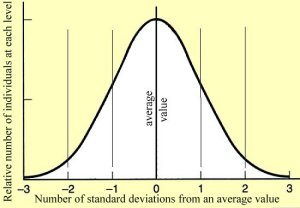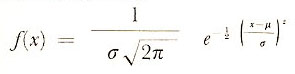A# normal distributionIn statistics, a normal distribution is an ideal distribution which is symmetrical about its mean. It can be written in the form:where f(x) is the relative frequency, σ is the standard deviation, μ is the mean, and π and e have their usual values. Note that in a normal distribution the mean, median, and mode coincide. The characteristic bell-shaped curve shown when f(x) is plotted against x is called the normal curve. The perfect bell curve or normal distribution only occurs when variables are perfectly random.

The normal distribution is also known as the Gaussian distribution for Karl Gauss.